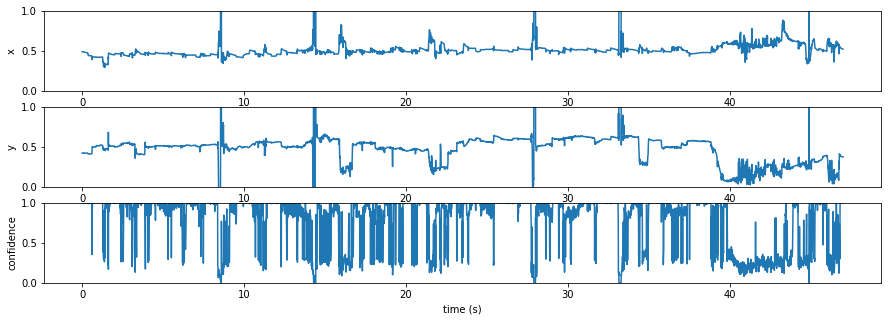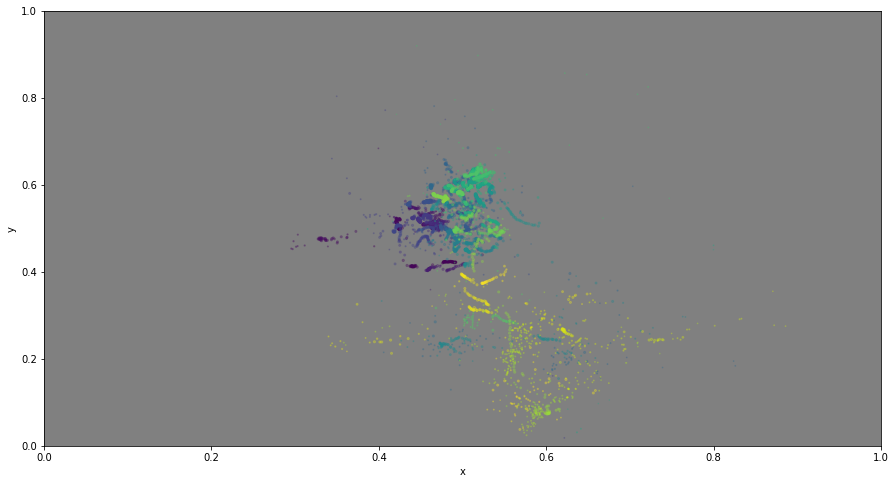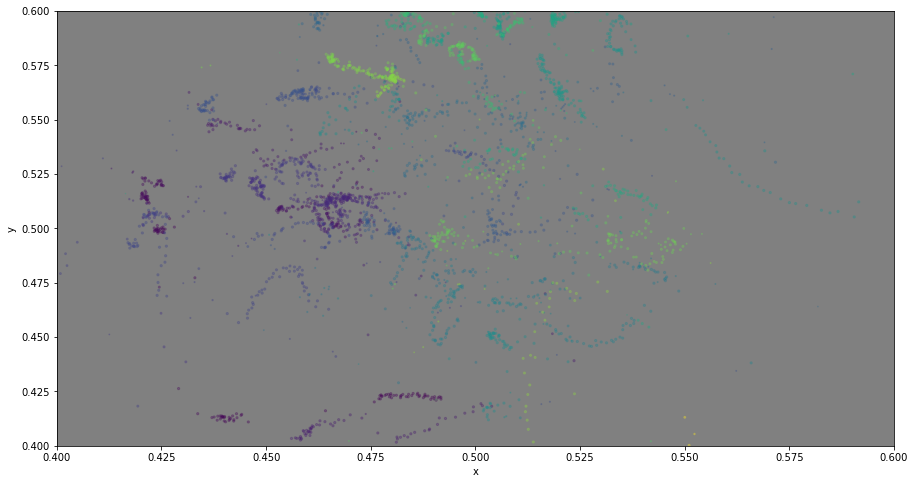# accessing the data from a pupil recording

I am experimenting with the pupil eyetracker and could set it up (almost) smoothly on a macOS. There is an excellent documentation, and my first goal was to just record raw data and extract eye position.

In :
from IPython.display import HTML
HTML('<center><video controls autoplay loop src="https://laurentperrinet.github.io/sciblog/files/2017-12-13_pupil%20test_480.mp4" width=61.8%/></center>')

Out:

This video shows the world view (cranio-centric, from a head-mounted camera fixed on the frame) with overlaid the position of the (right) eye while I am configuring a text box. You see the eye fixating on the screen then jumping somewhere else on the screen (saccades) or on the keyboard / hands. Note that the screen itself shows the world view, such that this generates an self-reccurrent pattern.

For this, I could use the capture script and I will demonstrate here how to extract the raw data in a few lines of python code.

In particular, we will use existing examples and rely on the documentation of the pupil's data format. Let's first access the data:

In :
import os
home = os.environ['HOME']

In :
path = os.path.join(home, 'science/pupil/pupil/recordings/2017_12_13/003')

In :
!ls {path}

depth.mp4            eye0_timestamps.npy  user_info.csv
depth_timestamps.npy info.csv             world.intrinsics
exports              offline_data         world.mp4
eye0.mp4             pupil_data           world_timestamps.npy


We can have a look at the meta-data:

In :
import pandas as pd
df

Out:
key value
0 Recording Name 2017_12_13
1 Start Date 13.12.2017
2 Start Time 11:33:13
3 Duration Time 00:00:47
4 World Camera Frames 2048
5 World Camera Resolution 640x480
6 Capture Software Version 1.2.7
7 Data Format Version 1.2.7
8 System Info User: laurentperrinet, Platform: Darwin, Machi...

Great. Raw data is stored in the pupil_data file. To access it, we will use the following code:

pupil_data = pd.read_pickle(os.path.join(path, 'pupil_data'))import pickle with open(os.path.join(path, 'pupil_data'), 'rb') as f: pupil_data = pickle.load(f, encoding='bytes')
In :
import msgpack


msgpack is a good replacement for pickle, check out:

help(msgpack)
In :
with open(os.path.join(path, 'pupil_data'), 'rb') as fh:
pupil_data = msgpack.unpack(fh, encoding='utf-8')


There is certainly a way to use directly

from file_methods import load_object l = load_object(os.path.join(path, 'pupil_data'))

In the meanwhile, we have all of our data:

In :
pupil_data.keys()

Out:
dict_keys(['pupil_positions', 'gaze_positions', 'notifications', 'fixations'])
In :
print('number of recording points: ', len(pupil_data['gaze_positions']))

number of recording points:  4943


Let's scrutinize one gaze position:

In :
one_gp = pupil_data['gaze_positions']
one_gp

Out:
{'base_data': [{'circle_3d': {'center': [-3.2808202989993225,
-2.8132283347674063,
24.500154048470392],
'normal': [-0.6232148623038679, -0.42114559533789575, -0.6589686054214271],
'confidence': 1.0,
'diameter': 77.76965434697944,
'diameter_3d': 3.0692721170585555,
'ellipse': {'angle': 34.685331501923365,
'axes': [41.029194035818, 77.76965434697944],
'center': [237.79220473012253, 169.32675966117165]},
'id': 0,
'method': '3d c++',
'model_birth_timestamp': 700523.227845,
'model_confidence': 0.6947833179596574,
'model_id': 1,
'norm_pos': [0.37155031989081644, 0.647235917372559],
'phi': -2.3283166698509525,
'projected_sphere': {'angle': 90.0,
'axes': [459.14904487414054, 459.14904487414054],
'center': [400.30819161037704, 282.86383630445124]},
'sphere': {'center': [4.197758048647093,
2.2405188092873427,
32.40777731352752],
'theta': 1.1360883069651921,
'timestamp': 700622.984498,
'topic': 'pupil'}],
'confidence': 1.0,
'eye_center_3d': [164.06609886548287, -165.38775347354547, 104.3377738743244],
'gaze_normal_3d': [-0.33378842343105425,
0.3708671791215458,
0.8666272692650739],
'gaze_point_3d': [-2.828112850044235, 20.045836087227453, 537.6514085068613],
'norm_pos': [0.4917810569110818, 0.42232484123674774],
'timestamp': 700622.984498,
'topic': 'gaze.2d.2'}

One may thus quickly extract data points as:

In :
import numpy as np
gaze_data = np.array([(one_gp['timestamp'], one_gp['norm_pos'], one_gp['norm_pos'], one_gp['confidence']) for one_gp in pupil_data['gaze_positions']])
gaze_data[:, 0] -= gaze_data[:, 0].min()


To finish, let's plot that data as a function of time (with an evaluation of its confidence):

In :
import matplotlib.pyplot as plt
%matplotlib inline

In :
fig, axs = plt.subplots(3, 1, figsize=(15, 5))
for i in range(3):
axs[i].plot(gaze_data[:, 0], gaze_data[:, i+1])
axs[i].set_ylim(0, 1)

axs.set_ylabel('x')
axs.set_ylabel('y')
axs.set_ylabel('confidence')
axs.set_xlabel('time (s)');... or in space:

In :
fig, ax = plt.subplots(1, 1, figsize=(15, 8), subplot_kw=dict(facecolor='gray'))
ax.scatter(gaze_data[:, 1], gaze_data[:, 2], c=gaze_data[:, 0], s=gaze_data[:, -1]*5, alpha=.3)
ax.set_xlim(0, 1)
ax.set_ylim(0, 1)

ax.set_xlabel('x')
ax.set_ylabel('y');In :
fig, ax = plt.subplots(1, 1, figsize=(15, 8), subplot_kw=dict(facecolor='gray'))
ax.scatter(gaze_data[:, 1], gaze_data[:, 2], c=gaze_data[:, 0], s=gaze_data[:, -1]*5, alpha=.3)
ax.set_xlim(.4, .6)
ax.set_ylim(.4, .6)

ax.set_xlabel('x')
ax.set_ylabel('y');